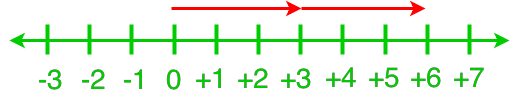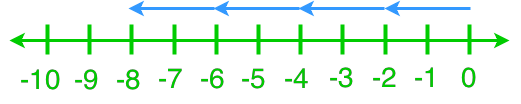# Multiplying integers#### All in One Place

Everything you need for JC, LC, and college level maths and science classes.#### Learn with Ease

We’ve mastered the national curriculum so that you can revise with confidence.#### Instant Help

0/14
##### Examples
###### Lessons
1. Understanding Multiplication Using a Number Line
Write the multiplication statement for each number line.

1.2.2. Multiplication of 1-digit Integers
Use a number line to find the product.
1. (+4) × (+4)
2. (+3) × (-8)
3. (-6) × (-2)
3. Multiplication of 2-digit Integers
Estimate. Then calculate.
1. (+18) × (-33)
2. (-21) × (+46)
3. (-37) × (-19)
4. Multiplication of Integers With Different Digits
Solve the following expressions.
1. (+36) × (-5)
2. (-86) × 0
3. (+167) × (+54)
4. (-245) × (-119)
5. Leo has to read 15 books during the school year. If each book has 334 pages, how many pages in total does Leo have to read?
1. Annie had 50 ml of yogurt every morning for the last 7 days. If there is 650 ml of yogurt left in the case, how much yogurt was there 7 days ago?
0%
##### Practice
###### Topic Notes
Continue from the previous section, we will practice more on multiplication of positive and negative integers. We will look at questions involving multiplying integers and number lines. In addition, we will learn how to estimate double digit integer multiplication.
• Any number multiplied by 0 will equal to 0